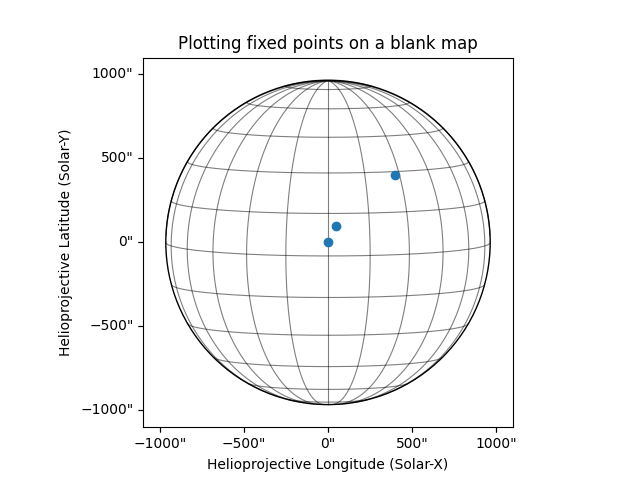# Plot positions on a blank map¶

This example showcases how to plot positions on a blank map. It is often useful to plot coordinate positions of events on a blank helioprojective coordinate map. In this example, we create an empty map with a WCS defined by a helioprojective frame as observed from Earth at a certain time, and show how you can plot different coordinates on it.

```import matplotlib.pyplot as plt
import numpy as np

import astropy.units as u
from astropy.coordinates import SkyCoord

import sunpy.map
from sunpy.coordinates import frames
```

First we will create a blank map using with an array of zeros. Since there is no WCS information, we will need to construct a header to pass to Map.

```data = np.full((10, 10), np.nan)

skycoord = SkyCoord(0*u.arcsec, 0*u.arcsec, obstime='2013-10-28',
observer='earth', frame=frames.Helioprojective)

# Scale set to the following for solar limb to be in the field of view

# Use sunpy.map.Map to create the blank map
```

Now we have constructed the map, we can plot it and mark important locations to it. Initialize the plot and add the map to it

```fig = plt.figure()
blank_map.plot(axes=ax)
blank_map.draw_limb(axes=ax, color="k")
blank_map.draw_grid(axes=ax, color="k")
```
```<CoordinatesMap with 2 world coordinates:

index aliases    type   unit    wrap   format_unit visible
----- ------- --------- ---- --------- ----------- -------
0     lon longitude  deg 180.0 deg         deg     yes
1     lat  latitude  deg      None         deg     yes

>
```

Coordinates that are being plotted - (0, 0), (50, 100) and (400, 400).

```xc = [0, 50, 400] * u.arcsec
yc = [0, 100, 400] * u.arcsec
```

Place and mark coordinates on the plot.

```coords = SkyCoord(xc, yc, frame=blank_map.coordinate_frame)
p = ax.plot_coord(coords, 'o')
# Set title.
ax.set_title('Plotting fixed points on a blank map')

plt.show()
```Total running time of the script: ( 0 minutes 0.971 seconds)

Gallery generated by Sphinx-Gallery